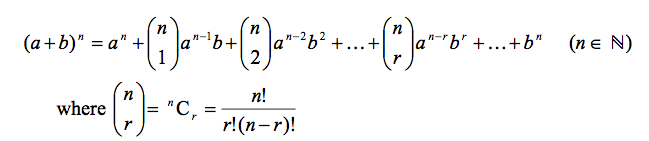Ah, the binomial expansion. The scourge of my A-level: the sum that was always wider than the paper, and always had one more minus sign than I’d allowed for. A crazy, pointless exercise in arithmetic, if you ask me, only really useful for finding square roots in your head (of which more another time).

### What is the binomial expansion?

Let’s say you have to work out something like $(2 - 3x)^7$. The naive way of doing that is to write out $(2-3x)$ seven times and multiply all of the brackets together - which takes weeks, of course.

There’s a simpler way - the binomial expansion - which tells you how to work it out in many fewer steps. It’s given to you in the formula book as:You’re allowed to recoil in horror, but it does make things a bit simpler… but it’s still a mess. In this article, I’ll show you how to break it down into easy bits - and lay it out so you have plenty of paper.

### Making a table

The most useful bit of the formula is actually the most horrible bit. It’s the bit between the dots - the $^nC_r a^{n-r}b^r$ bit. That actually tells you everything you need to know (maths is like that: a language for expressing complicated ideas very concisely).

What it tells you is that there are three parts to each term in the binomial expansion:

• A number from Pascal’s triangle (the $^nC_r$);
• A power of the first thing in the bracket ($a^{n-r}$); and
• A power of the second thing in the bracket ($b^r$).

These are going to be the first three columns of the table - I like to call them C, A and B - and we’ll have a fourth column as well, which is CAB - the three things multiplied together.

### The first column

The first column is the numbers from one of the rows in Pascal’s triangle - the one that has the second number being the power you’re putting the bracket to (I’ll call that $n$). If $n$ is below about six, it’s easy enough to draw out Pascal’s triangle if you know what that is; any higher than that and it gets a bit error-prone.

In any event, you can get the number out of your calculator using the $^nC_r$ button - on my calculator it’s shift and ÷. The top row is $^nC_0$, the next one is $^nC_1$ and so on, all the way up to $n$.

(If you’re studying C4 and have your hand up to say ‘it doesn’t work with negative or fractional powers!’ - yes, I know. There’s a Part II coming soon. Just hold tight, get used to this way and it’ll just need a small change.)

For the example I mentioned earlier, $(2 - 3x)^7$, your $n$ is 7. That makes the first column 1, 7, 21, 35, 35, 21, 7, 1.

### The second column

The second column is the one corresponding to $a^{n-r}$. What that means is, you take the first Thing in the bracket (here, it’s $2$), start by putting that to the $n$th power, and drop the power by 1 each time - effectively dividing by the Thing. So, you start from $2^7 = 128$ and halve it each time. (When $n$ is a positive integer, this will always end at 1). Here, you get 128, 64, 32, 16, 8, 4, 2, 1 in the second column.

### The third column

The third column corresponds to $b^r$. This means, take the second Thing in the bracket (including any minus sign - here it’s $-3x$), start by putting it to the zeroth power and add one to the power each time - same as multiplying by the Thing. The third column here would be $1,\ -3x,\ 9x^2,\ -27x^3,\ 81x^4,\ -243x^5, 729x^6,\ -2187x^7$.

### The final columns

Lastly, for the CAB column, you just multiply the three Things in each row together. Here’s how it looks:

 C A B CAB 1 128 $1$ $128$ 7 64 $-3x$ $-1344x$ 21 32 $9x^2$ $6048x^2$ 35 16 $-27x^3$ $-15120x^3$ 35 8 $81x^4$ $22680x^4$ 21 4 $-243x^5$ $-20412x^5$ 7 2 $729x^6$ $10206x^6$ 1 1 $-2189x^7$ $-2189x^7$

… and the only thing that remains to do is to write out the whole thing in one line (if you can fit it): $(2-3x)^7 = 128 - 1344x + 6048x^2 - 15,120x^3 + 22,680x^4 - 20412x^5+10206x^6 - 2189x^7$. Job done!

(You’d be really unlucky to get anything that big in an exam - the worst case scenario for the ‘whole thing’ is $x^5$, or you might be asked for the first three or four terms of a bigger power. In that case, you just end the table after four rows, easy!)